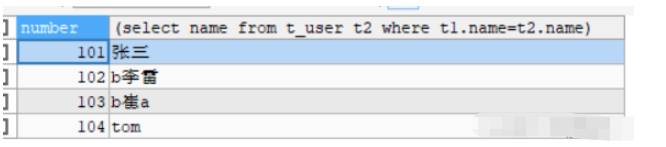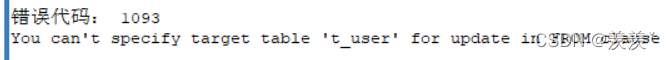# mysql子查询怎么使用

```-- 子查询
-- 查询的条件来自于另一查询的结果
SELECT * FROM t_user WHERE number=(SELECT number FROM t_user WHERE NAME='张三')```

• 标量子查询（结果集只有一行一列）

• 列子查询（结果集只有一列多行）

• 行子查询（结果集有一行多列）（较少）

• 表子查询（结果集一般为多行多列）select后面：仅仅支持标量子查询

```-- select语句后面,将t1查询出的结果作为子查询的条件
SELECT t1.number,
(SELECT NAME FROM t_user t2 WHERE t1.name = t2.name)
FROM t_user t1```insert into , update和 delete后面 :

insert into用来为表中插入数据 , 所以后面是可以跟列子查询和表子查询的

```-- insert into 后跟子查询
INSERT INTO t_user(number,NAME,age,birthday,weight,sex,opertime)
SELECT number,NAME,age,birthday,weight,sex,NOW() FROM t_user WHERE id=3```

UPDATE t_user SET NAME='abc' WHERE number=(SELECT number FROM t_user WHERE weight=110)

DELETE FROM t_user WHERE id=(SELECT id FROM t_user WHERE id=7)mysql的底层机制使得我们在操作此表的时候是不能去改变此表结构的

where 后面 : 可跟标量子查询, 列子查询, 行子查询

```-- where后跟标量子查询
-- 查询体重最大的人信息
SELECT * FROM t_user WHERE weight=(SELECT MAX(weight) FROM t_user)
-- where后跟列子查询
-- 查询体重大于或等于130的人信息
SELECT * FROM t_user WHERE weight IN (SELECT weight FROM t_user WHERE weight>=130)
-- where后跟行子查询
-- 查询年龄最大,体重最大的人的信息
SELECT * FROM t_user
WHERE (age,weight) = (SELECT MAX(age),MAX(weight) FROM t_user)```

from 后面 : 可跟表子查询

```-- 表子查询
SELECT t.age FROM (SELECT age,weight FROM t_user)t```